# Chaos in the frequency domain

Solutions to the non-linear differential equation

x ″ + 0.25x ′ + x(x² – 1) = 0.3 cos t

are chaotic. It’s more common to see plots of chaotic systems in the time domain, so I wanted to write a post looking at the power spectrum in the frequency domain.

The following plot was created by solving the equation above over the time interval [0, 256] at 1024 points, i.e. sampling the solution at 4 Hz. I then took the FFT and multiplied it by its conjugate to get the power spectrum. Then I took the log base 10 and multiplied by 10 to convert to decibels.By contrast, if we look at the linear equation

x ″ + 0.25x ′ + x = 0.3 cos t

and compute the power spectrum, we get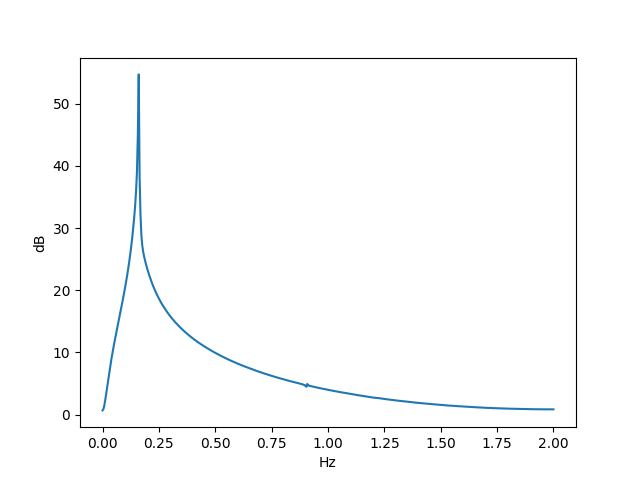As is often the case, a small change to the form of a differential equation made a huge change in its behavior.

There’s a spike at 1/2π Hz because the steady-state solution is

x(t) = 1.2 sin(t).

The power spectrum is more than just a spike because there is also an exponentially decaying transient component to the solution.

For more on steady-state and transient components of the solution, see Damped, driven oscillations.

# The tent map

Yesterday I said that Lyapunov exponents can’t be calculated exactly except in the case of toy problems. One such toy model is the tent map.The graph of function on the right hand side looks a tent. It’s zero at x = 0 and at x = 1 and rises to a height of r in the middle. The Lyapunov exponent of this system has been calculated  to be

λ = log 2r.

For r < 1/2 the Lyapunov exponent is negative and the system is stable.

For r > 1/2 the Lyapunov exponent is positive and the system is chaotic. The larger r is, the faster uncertainty in the future values of x grows. In fact the uncertainty grows in proportion to (2r)n.

Suppose r = 1 and we know the floating point representation of x0. Suppose we compute the iterations of the tent map exactly. There is no rounding error, and the only uncertainty comes from the initial uncertainty in x0. Assuming we’re using an IEEE 754 double precision number, our initial uncertainty is 2-53. (See details here.)

We lose one bit of precision in the value of x at each iteration. After 53 iterations, we’ve lost all information: the true value of x53 could be anywhere in [0, 1], and our calculated value gives us no clue where in the interval x actually is.

Here’s a cobweb plot of the iterations starting with x0 = 4 – π.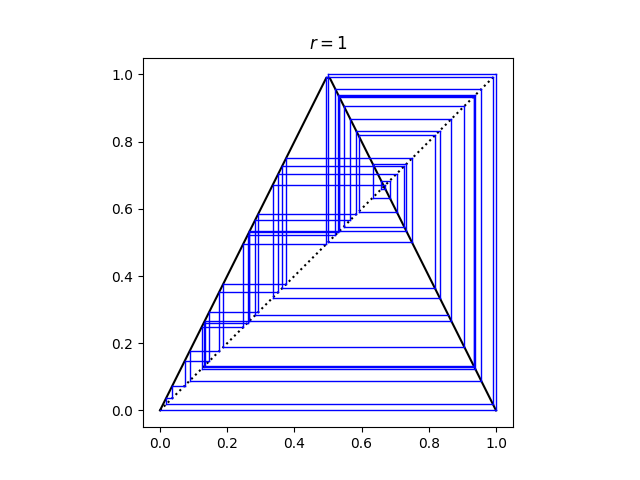The solid black lines are the tent map. Vertical lines connect each iterate to its next value. Horizontal lines bring each iterate back to the line y = x to start the next iteration.

Incidentally, after 49 iterations the computed value x reaches 0 and stays there. This would not happen if we started with exactly x0 = 4 – π and carried out each iteration in exact arithmetic because then all the values of x are irrational.

Related posts: Chaos and dynamical systems

 Lichtenberg and Lieberman. Regular Stochastic Motion. Springer-Verlag 1983.

# A different view of the Lorenz system

The Lorenz system is a canonical example of chaos. Small changes in initial conditions eventually lead to huge changes in the solutions.

And yet discussions of the Lorenz system don’t simply show this. Instead, they show trajectories of the system, which make beautiful images, but do not demonstrate the effect of small changes to initial conditions. Or they demonstrate it in two or three dimensions where it’s harder to see.

If you’ve seen the Lorenz system before, this is probably the image that comes to mind.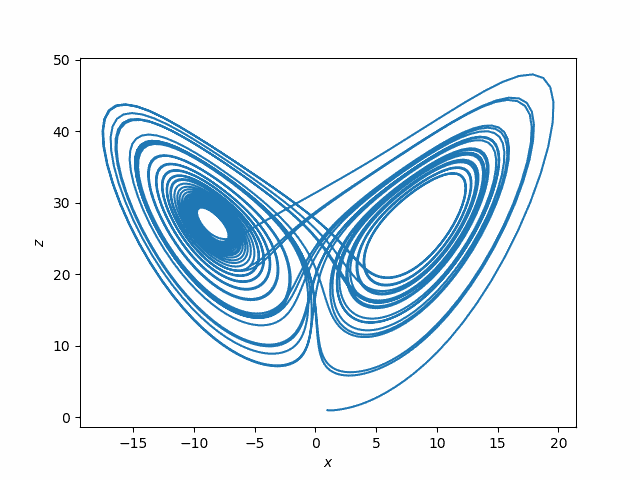This plots (x(t), z(t)) for the solutions to the system of differential equations

x‘= σ(yx)
y‘ = x(ρ – z) – y
z‘ = xy – βz

where σ = 10, ρ = 28, β = 8/3. You could use other values of these parameters, but these were the values originally used by Edward Lorenz in 1963.

The following plots, while not nearly as attractive, are more informative regarding sensitive dependence on initial conditions.In this plot, x1 is the x-component of the solution to the Lorenz system with initial condition

(1, 1, 1)

and x2 the x-component corresponding to initial condition

(1, 1, 1.00001).

The top plot is x1 and the bottom plot is x1x2.

Notice first how erratic the x component is. That might not be apparent from looking at plots such as the plot of (x, z) above.

Next, notice that for two solutions that start off slightly different in the z component, the solutions are nearly identical at first: the difference between the two solutions is zero as far as the eye can tell. But soon the difference between the two solutions has about the same magnitude as the solutions themselves.

Below is the Python code used to make the two plots.

    from scipy import linspace
from scipy.integrate import solve_ivp
import matplotlib.pyplot as plt

def lorenz(t, xyz):
x, y, z = xyz
s, r, b = 10, 28, 8/3. # parameters Lorentz used
return [s*(y-x), x*(r-z) - y, x*y - b*z]

a, b = 0, 40
t = linspace(a, b, 4000)

sol1 = solve_ivp(lorenz, [a, b], [1,1,1], t_eval=t)
sol2 = solve_ivp(lorenz, [a, b], [1,1,1.00001], t_eval=t)

plt.plot(sol1.y, sol1.y)
plt.xlabel("$x$")
plt.ylabel("$z$")
plt.savefig("lorenz_xz.png")
plt.close()

plt.subplot(211)
plt.plot(sol1.t, sol1.y)
plt.xlabel("$t$")
plt.ylabel("$x_1(t)$")
plt.subplot(212)
plt.plot(sol1.t, sol1.y - sol2.y)
plt.ylabel("$x_1(t) - x_2(t)$")
plt.xlabel("$t$")
plt.savefig("lorenz_x.png")


One important thing to note about the Lorenz system is that it was not contrived to show chaos. Meteorologist and mathematician Edward Lorenz was lead to the system of differential equations that bears his name in the course of his work modeling weather. Lorenz understandably assumed that small changes in initial conditions would lead to small changes in the solutions until numerical solutions convinced him otherwise. Chaos was a shocking discovery, not his goal.

# Logistic bifurcation diagram in detail

The image below is famous. I’ve seen it many times, but rarely with a detailed explanation. If you’ve ever seen this image but weren’t sure exactly what it means, this post is for you.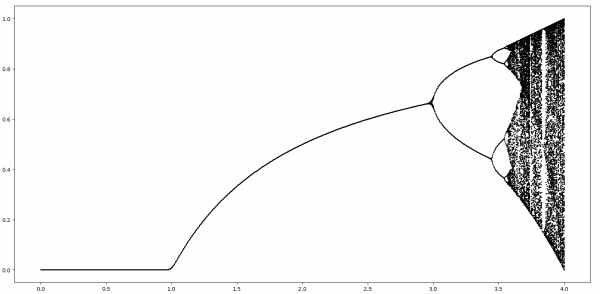This complex image comes from iterating a simple function

f(x) = r x(1 – x)

known as the logistic map. The iterates of the function can behave differently for different values of the parameter r.

We’ll start by fixing a value of r, with 0 ≤ r < 1. For any starting point 0 ≤ x ≤ 1, f(x) is going to be smaller than x by at least a factor of r, i.e.

0 ≤ f(x) ≤ rx.

Every time we apply f the result decreases by a factor of r.

0 ≤ f( f(x) ) ≤ r²x.

As we do apply f more and more times, the result converges to 0.

For r ≥ 1 it’s not as clear what will happen as we iterate f, so let’s look at a little bit of code to help see what’s going on.

    def f(x, r):
return r*x*(1-x)

def iter(x, r, n):
for _ in range(n):
x = f(x, r)
return x


We can see that for 1 ≤ r ≤ 3, and 0 ≤ x ≤ 1, the iterations of f converge to a fixed point, and the value of that fixed point increases with r.

    >>> iter(0.1, 1.1, 100)
0.09090930810626502

# more iterations have little effect
>>> iter(0.1, 1.1, 200)
0.09090909091486

# different starting point has little effect
>>> iter(0.2, 1.1, 200)
0.0909090909410433

# increasing r increases the fixed point
>>> iter(0.2, 1.2, 200)
0.16666666666666674


Incidentally, for 1 ≤ r ≤ 3, the fixed point equals (r – 1)/r. 

When r is a little bigger than 3, things get more interesting. Instead of a single fixed point, the iterates alternate between a pair of points, an attractor consisting of two points.

    >>> iter(0.2, 3.1, 200)
0.7645665199585945

>>> iter(0.2, 3.1, 201)
0.5580141252026958

>>> iter(0.2, 3.1, 202)
0.7645665199585945


How can we write code to detect an attractor? We can look at the set of points when we get, say when we iterate 1000, then 1001, etc. up to 1009 times. If this set has less than 10 elements, the iterates must have returned to one of the values. We’ll round the elements in our set to four digits so we actually get repeated values, not just values that differ by an extremely small amount.

    def attractors(x, r):
return {round(iter(x, r, n), 4) for n in range(1000,1010)}


This is crude but it’ll do for now. We’ll make it more efficient, and we’ll handle the possibility of more than 10 points in an attractor.

Somewhere around r = 3.5 we get not just two points but four points.

    >>> attractors(0.1, 3.5)
{0.3828, 0.5009, 0.8269, 0.875}


As r gets larger the number of points keeps doubling  until chaos sets in.

The function attractors is simple but not very efficient. After doing 1000 iterations, it starts over from scratch to do 1001 iterations etc. And it assumes there are no more than 10 fixed points. The following revision speeds things up significantly.

    def attractors2(x, r):
x = iter(x, r, 100)
x0 = round(x, 4)
ts = {x0}
for c in range(1000):
x = f(x, r)
xr = round(x, 4)
if xr in ts:
return ts
else:


Notice we put a cap of 1000 on the number of points in the attractor for a given r. For some values of r and x, there is no finite set of attracting points.

Finally, here’s the code that produced the image above.

    import numpy as np
import matplotlib.pyplot as plt
rs = np.linspace(0, 4, 1000)
for r in rs:
ts = attractors2(0.1, r)
for t in ts:
plt.plot(r, t, "ko", markersize=1)
plt.show()


Update: See this post for graphs showing the trajectory of points over time for varying values of r.

## More dynamical system posts

 Why is this only for 1 ≤ r ≤ 3? Shouldn’t (r – 1)/r be a fixed point for larger r as well? It is, but it’s not a stable fixed point. If x is ever the slightest bit different from (r – 1)/r the iterates will diverge from this point. This post has glossed over some fine points, such as what could happen on a set of measure zero for r > 3.

 The spacing between bifurcations decreases roughly geometrically until the system becomes chaotic. The ratio of one spacing to the next reaches a limit known as Feigenbaum’s constant, approximately 4.669. Playing with the code in this post and trying to estimate this constant directly will not get you very far. Feigenbaum’s constant has been computed to many decimal places, but by using indirect methods.

# Exact chaos

Pick a number x between 0 and 1. Then repeatedly replace x with 4x(1-x). For almost all starting values of x, the result exhibits chaos. Two people could play this game with starting values very close together, and eventually their sequences will diverge.

It’s somewhat surprising that the iterative process described above can be written down in closed form. Starting from a value x0, the value after n iterations is

sin( 2n arcsin( √ x0 ) )2.

Now suppose two people start with the same initial value. One repeatedly applies 4x(1-x) and the other uses the formula above. If both carried out their calculations exactly, both would produce the same output at every step. But what if both used a computer?

The two approaches correspond to the Python functions f and g below. Because both functions are executed in finite precision arithmetic, both have errors, but they have different errors. Suppose we want to look at the difference between the two functions as we increase n.

from scipy import arcsin, sin, sqrt, linspace
from matplotlib import pyplot as plt

def f(x0, n):
x = x0
for _ in range(n):
x = 4*x*(1-x)
return x

def g(x0, n):
return sin(2.0**n * arcsin(sqrt(x0)))**2

n = 40
x = linspace(0, 1, 100)
plt.plot(x, f(x, n) - g(x, n))
plt.ylim(-1, 1)
plt.show()

When we run the code, nothing exciting happens. The difference is a flat line.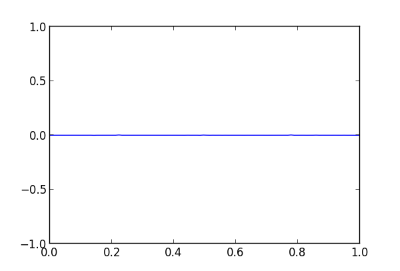Next we increase n to 45 and we start to see the methods diverge.The divergence is large when n is 50.And the two functions are nearly completely uncorrelated when n is 55.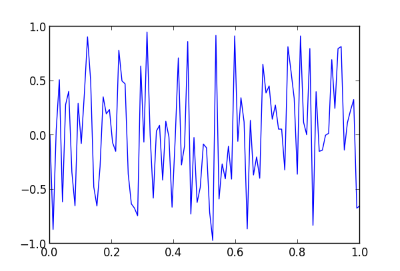Update

So which function is more accurate, f or g? As noted in the comments, the two functions have different kinds of numerical errors. The former accumulates arithmetic precision error at each iteration. The latter shifts noisy bits into significance by multiplying by 2^n. Apparently both about the same overall error, though they have different distributions of error.

I recomputed g using 100-digit precision with mpmath and used the results as the standard to evaluate the output of f and g in ordinary precision. Here’s a plot of the errors when n = 45, first with fand then with g.The average absolute errors of f and g are 0.0024 and 0.0015 respectively.